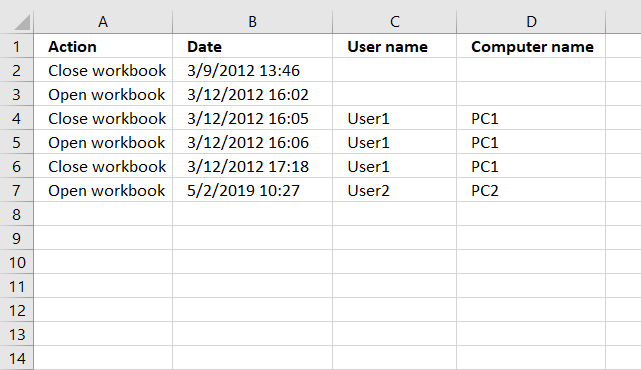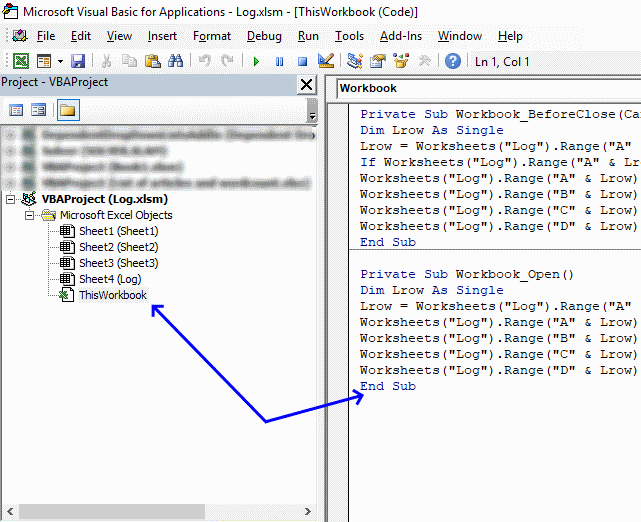Author: Oscar Cronquist Article last updated on May 02, 2019This article demonstrates how to automatically create log entries when a workbook opens or closes using event code. Column A contains the action, Open or Close, column B contains the date and time.

Column C contains the user name and column D contains the computer name. Do not paste the code below to a regular module, that won't work. There are detailed instructions below.

VBA code

```'This event code begins right before the user closes the workbook.
Private Sub Workbook_BeforeClose(Cancel As Boolean)

'Dimension variable and declare data type
Dim Lrow As Single

'Save the row of the first empty cell in column A to variable Lrow.
Lrow = Worksheets("Log").Range("A" & Rows.Count).End(xlUp).Row + 1

'Check if cell above equals text value "Close Workbook",
'if true then withdraw value in Lrow with 1 and then save the result to Lrow
If Worksheets("Log").Range("A" & Lrow - 1).Value = "Close workbook" Then Lrow = Lrow - 1

'Save text value "Close Workbook" to cell
Worksheets("Log").Range("A" & Lrow).Value = "Close workbook"

'Save date and time to the corresponding cell in column B.
Worksheets("Log").Range("B" & Lrow).Value = Now
End Sub
```
```'This event code starts when the workbook is opened.
Private Sub Workbook_Open()

'Dimension variable and declare data type
Dim Lrow As Single

'Save the row of the first empty cell in column A to variable Lrow.
Lrow = Worksheets("Log").Range("A" & Rows.Count).End(xlUp).Row + 1

'Save text value "Open Workbook" to the first empty cell
Worksheets("Log").Range("A" & Lrow).Value = "Open workbook"

Save date and time to the corresponding cell in column B.
Worksheets("Log").Range("B" & Lrow).Value = Now
End Sub
```

### Where to copy code?1. Go to tab "Developer" on the ribbon and click "Visual Basic" button to open the Visual Basic Editor or press shortcut keys Alt+F11
2. Double click "This workbook" in the project explorer.
3. Paste code to workbook module.
4. Return to Excel.
5. Save your workbook as an *.xslm file in order to save the code as well.

### Download Excel file

* You will also get a weekly newsletter, unsubscribe anytime!